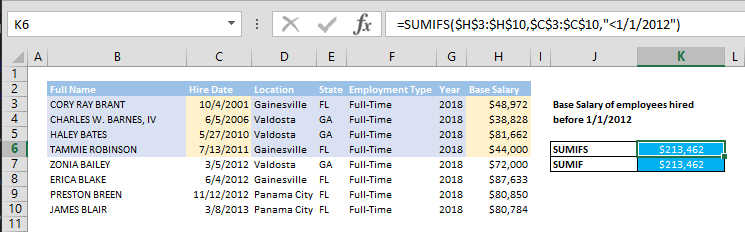## How to create an Excel summary table using UNIQUE and SUMIFS

SUMIFS like other …IF or …IFS function are great tools to aggregate data based on a set of conditions. The downside of this approach is that the criteria must be supplied manually, especially when you need to create a summary table. In this guide, we’re going to show...

## Function: SUMIF

SUMIF function can sum up the values in a range of cells that match a single criteria. In this guide, we’re going to show you how to use the SUMIF function and also go over some tips and error handling methods. Supported versions All Excel versions Syntax SUMIF(range,...## How to SUM 2d ranges with SUMPRODUCT

Summing values by a condition may sound easy. If not, please see Crunch Data Even Faster with the SUMIFS Function article as well. You can easily sum values by condition(s) with SUMIF and SUMIFS functions; with a limitation. The sum range and criteria ranges should...## How to SUM values if date is less than

How much did you spend until last year, or what is the total of X until Y? SUMIF and SUMIFS functions can help you to sum values in your data tables. Syntax =SUMIFS(values to sum range, date range,## Extract Data Quickly From Tables with Excel's SUMIF Function

SUMIF function is, in a way, a combination of SUM and IF statements. The formula SUMs up the values of a range of cells, IF certain criteria is met. In other words, data that pass the logic test gets to be added up (or another column of your choice, that corresponds...